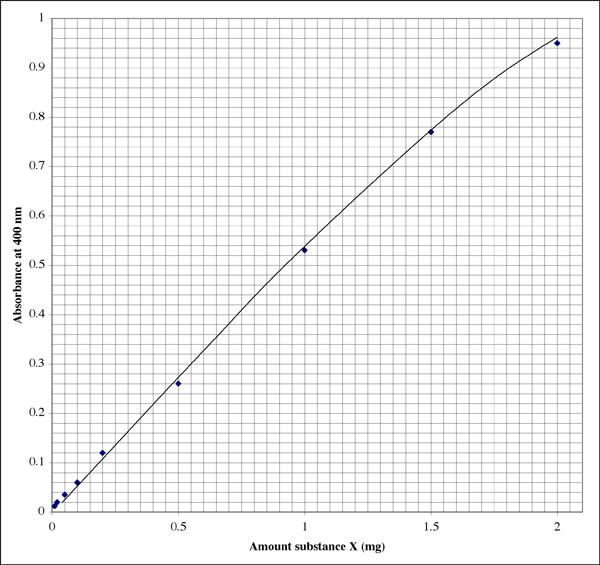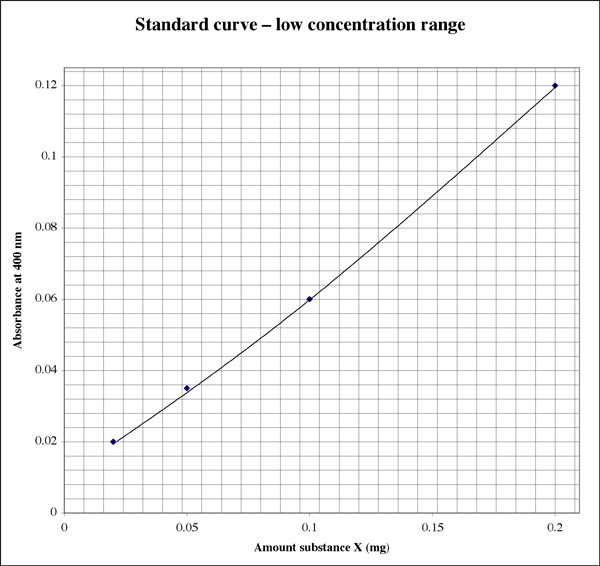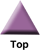### Home

Laboratory
Studies

Recordkeeping, Writing,
& Data Analysis

Laboratory
Methods

Overview
Microscope studies

Flagella experiment
Laboratory math
Blood fractionation
Gel electrophoresis
Protein gel analysis
Mitochondria
Concepts/ theory
Overview
Keeping a lab notebook
Writing research papers
Dimensions & units
Using figures (graphs)
Examples of graphs
Experimental error
Representing error
Applying statistics
Overview
Principles of microscopy

Solutions & dilutions
Protein assays
Spectrophotometry
Fractionation & centrifugation

# Colorimetric Assays

Here is a description of how one sets up and runs a colorimetric assay to determine the concentration of a substance that is in solution.

## General approach

We cannot put material under a microscope and count the number of molecules per unit volume the way we can count number of cells per unit volume. We must find something that we can measure that is proportional to the concentration of the substance of interest. The measurement most commonly used in assays is absorbance of light. Beer's Law tells us that if a solute absorbs light of a particular wavelength, the absorbance is directly proportional to the concentration of substance in solution. A device called a spectrophotometer is used to measure and display and/or record absorbance in quantifiable units. Often the substance by itself does not absorb light so as to allow for a practical assay. We may have to employ one or more reagents to produce colored compounds in proportion to the concentration of unknown.

Measuring absorbance of light by a sample tells us very little unless we have a standard for comparison. For example, if sample X shows absorbance of 0.5, what is the actual concentration of X? If we have a sample of known concentration, and that sample also gives absorbance of 0.5, then we are reasonably sure that the substance has that same concentration. Suppose that you have a number of samples, and their concentrations vary. It would be useful to have a number of standards that span the full range of likely concentrations of our unknown. That's where a standard curve comes into it. We prepare a series of standards of known concentration of X, ranging from low to high concentration. We run the assay and plot absorbance versus concentration for each standard. Using this standard curve we can read the concentration for an unknown given its absorbance reading.

## Controls

When we run an assay we must ensure that only the substance we are assaying is responsible for absorbance of light in the wavelength range of interest. All conditions under which standards and unknowns are prepared should be kept identical. If solutes in the sample buffers affect absorbance, then we have a problem. We won't obtain accurate results if we vary the volumes in which we prepare and assay standards and unknowns. The timing of reading absorbance, temperature at which we keep the materials, and all other physical factors should be kept the same. Because it is not always practical to use identical buffers for all unknowns and standards, we need only ensure that none of the components of any of the buffers has a significant effect on absorbance.

When we use the same volume for all standards and unknowns we simplify the analysis considerably. The standard curve can plot absorbance versus amount of substance instead of concentration. It may be less confusing to work with amounts while doing an assay, especially if dilutions are required. As long as you know the original volume of sample that was used in an assay, determination of concentration is easy.

## Complication

All assays have limits. Amounts of substance below some minimum will be undetectable. Beyond some maximum amount or concentration an assay becomes saturated, that is, increases in amount or concentration do not affect absorbance. We generally try to work within the linear range of an assay, that is, where absorbance is directly proportional to concentration. Ideally, we would set up standards that encompass the entire useful range of an assay. That is, we optimize the range of the assay.

Often a sample is so concentrated that when you assay the prescribed volume of sample the result is off scale – the assay reagent is saturated. The solution then is to dilute the sample. For example, if the volume of each standard or sample is 1 ml, and 1 ml of your unknown gives a result that is off scale, you can add 0.1 ml sample to a test tube along with 0.9 ml buffer. If you read a concentration from the standard curve, then multiply the result by 10 to get the actual concentration in the sample. If you read an amount from the standard curve then simply divide that amount by 0.1 ml to get your concentration.

When samples are so concentrated that you cannot pipet a small enough amount accurately, you may have to conduct serial dilutions.

## Example: preparing a standard curve

We will set up a hypothetical assay to measure substance X. When X is mixed with assay reagent a complex is formed that absorbs light at wavelength 400 nm. Our spectrophotometer requires that we put 2 ml volume in each cuvette. A cuvette is a transparent vessel to be placed in a light path for measurement of absorbance. To get the right proportion of assay reagent to sample, we make our sample volume 0.5 ml and add 1.5 ml color reagent to each tube. Set up in this manner the assay can detect amounts of X of as little as 10 micrograms (µg) to as much as 2 milligrams (mg).

### Reference

To calibrate the spectrophotometer we need a reference tube that is identical in every respect to the standards and samples, except that it does not contain any substance X. With the light path blocked the spectrophotometer will be set to read infinite absorbance (no transmittance of light at all). With the reference tube in the light path we will set the spectrophotometer to read zero absorbance. That way, a sample containing X will give absorbance within that range. The reference tube is used to give us the maximum dynamic range.

For this hypothetical example the reference will contain 0.5 ml sample buffer and 1.5 ml color reagent.

### Standards

This example describes a hypothetical assay for illustration purposes only.

We want the best accuracy that we can get, and our range spans two orders of magnitude, so one way to set up the standard curve is with a logarithmic progression of standards. We need standards from 0.01 mg to 2 mg. Let's try amounts of 0.01, 0.02, 0.05, 0.1, 0.2, 0.5, 1, and 2 mg. The last gap is rather wide, so let's toss in one standard of, say, 1.5 mg. To prepare standards it is convenient to start with a concentrated stock solution of the substance. The largest amount that we need is 2 mg, in a volume of 0.5 ml. Just to give us a little "wiggle room" let's make a stock solution of 5 mg/ml substance X. The following table presents the calculations.

Table 1. Example of how to plan a standard curve. The concentration of protein in the stock solution was 5 mg/ml. This example is for illustration purposes only.

 amount of substance X (mg) volume of stock solution (µl) volume of buffer (µl) 0 (reference) 0 500 0.01 2 498* 0.02 4 496* 0.05 10 490 0.1 20 480 0.2 40 460 0.5 100 400 1 200 300 1.5 300 200 2 400 100

*It is common to use pipettors that give us volumes that are accurate to no more than 2 significant figures.

The volume of buffer is not as critical as the volume of stock solution. Errors in pipetting buffer affect total volume and, thus, concentration of the color reagent. Errors of less than 1% will not have a significant effect on the results. In fact, if the volume of color reagent far exceeds the volume of sample (not in this case) we would not even need to equalize volumes by adding buffer.

Some labs are not equipped with pipettors that go below 5 µl with accuracy. It may be necessary to conduct a serial dilution to get, say, 2 or 4 µl stock solution into an assay tube.

### Sample preparation

It helps to have a reasonable estimate of ranges of concentrations of sample that one can expect. Even with such an estimate it is good to prepare samples with a range of dilutions, in case a sample is so concentrated that its absorbance readings are out of range.

For the assay in the example, if we use 500 µl sample in an assay tube (the maximum volume), its concentration would have to be less than 4 mg/ml to give a readable absorbance. On the other hand, we would want that much if the sample was, say, ten times less concentrated. Knowing nothing about the concentration of a particular sample, we would load one tube with 500 µl to cover that range. Since the assay spans a wide range of concentrations, we can use 50 µl in a second assay tube. Now the sample can be as concentrated as 40 mg/ml and we will still have 4 mg or less in the assay tube, giving a readable result. To cover all of the bases we can assay a third tube with just 5 µl sample.

### Run the assay

When all of the standard and unknowns are ready we will have:

• 1 reference tube
• some number of standards that span the full range of the assay
• two or three assay tubes per sample representing a series of dilutions

It is time to conduct the procedure for color development, which may be as simple as adding a color reagent and letting the samples sit for a few minutes. When practical, treatment of each standard and sample should be timed so that absorbance is read following the same time interval for each tube. The instrument should be calibrated, then absorbances should be taken for each tube in order. A standard curve is obtained by plotting absorbance versus amount of substance X. If the relationship is clearly linear, a standard curve isn't even necessary. Amounts can be determined using interpolation. A curve should be constructed the first time an assay is used, to check for accuracy and linearity.

### Example of a standard curve

Here is what the plot might look like in a lab notebook (the student obviously has excellent handwriting). The relationship is not perfectly linear, rather it shows a typical extinction pattern.Since the range is so wide, for samples giving very low absorbance readings a student might want a second higher resolution plot.### Determine concentration of a sample

A concentration is an amount of something per unit volume. We typically report protein concentrations in milligrams per milliliter(mg/ml), although it is sometimes convenient to use micrograms/microliter (µg/µl) or perhaps even µg/ml (for very small concentrations). For an unknown, we divide amount of substance (from the standard curve) by the volume of sample used in the assay. Note that this volume is not the assay volume, nor is it the diluted sample volume. Divide by the volume of undiluted sample that you placed in the assay tube.

Let us suppose that you prepared three assay tubes for sample #1, containing 500 µl, 50 µl, and 5 µl sample, respectively. Suppose they gave absorbance readings of 0.86, 0.12, and 0.01, respectively. The last absorbance is off scale, of course. The intercept should be zero, but we cannot count on very low absorbances giving us sufficiently accurate readings.\

An absorbance of 0.86 corresponds with 1.7 mg substance X. The volume was 500 µl (0.5 ml), so we get a concentration of 3.4 mg/ml. Sounds good. Checking the other readable tube, absorbance of 0.12 indicates that the tube contained 0.20 mg substance X. The volume was 50 µl (0.050 ml). The concentration should be 0.20 mg/0.050 ml = 4.0 mg/ml. Which result do we use, or do we take an average?

I've found that using the one absorbance reading that falls closest to the middle of the sensitive range gives the most accurate results. In the example above the center is an asorbance of 0.5, corresponding to 0.1 mg of substance. The absorbance scale is logarithmic, so that even from a digital display the readings are more reliable at the low end of the scale. However, at very low absorbances one or more unknown factors, such as a defect in the sample tube or cuvette, will have a more profound effect on the absorbance value than at higher absorbances. At the upper end of the range the color reagent approaches saturation, so that not only do you have less resolution among absorbance readings, but the reagent is less sensitive to differences in protein concentration.